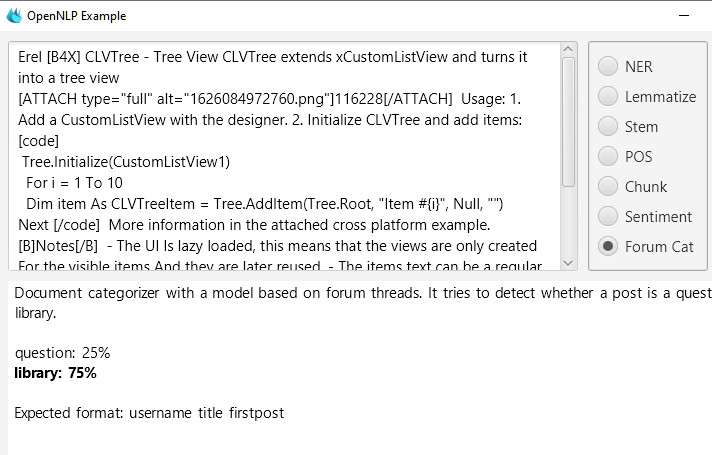# B4J Tutorial[NLP] Library or Question?It is quite similar.

In this tutorial we will try to detect whether the text belongs to a question thread or a library thread.
First step is to build the train and test data. The format, as explained here: https://opennlp.apache.org/docs/1.9.3/manual/opennlp.html#tools.doccat.training, is a text file where each line starts with the category, a space, and then the document text.

This is done with this code:
B4X:
``````#AdditionalJar: mysql-connector-java-5.1.38-bin
Sub Process_Globals
Private Const LIBRARY_B4A_ID = 29, LIBRARY_B4J_ID = 78, LIBRARY_B4I_ID = 64, QUESTIONS_ID = 26 As Int
Private sql As SQL
Private postsWithAttachments As B4XSet
End Sub

Sub AppStart (Args() As String)
Log("Hello world!!!")
Crawl
End Sub

Private Sub Crawl
postsWithAttachments.Initialize
Dim rs As ResultSet = sql.ExecQuery2("SELECT content_id FROM xf_attachment", Null)
Do While rs.NextRow
Loop
rs.Close
Dim rs As ResultSet = sql.ExecQuery2(\$"SELECT title, post_id, message, node_id, xf_post.reaction_score
(node_id = ? OR node_id = ? OR node_id = ? OR node_id = ?)"\$, _
Array(LIBRARY_B4A_ID, LIBRARY_B4I_ID, LIBRARY_B4J_ID, QUESTIONS_ID))

Dim train As List
train.Initialize
Dim evaluate As List
evaluate.Initialize
Dim counter As Int
Do While rs.NextRow
Dim doc As String = HandleDocument(rs)
IIf(counter Mod 3 < 2, train, evaluate).As(List).Add(doc)
counter = counter + 1
Log(counter)
Loop
rs.Close
sql.Close
Log("Size: " & evaluate.Size)
Log("Size: " & train.Size)
File.WriteList(File.DirApp, "train.txt", train)
File.WriteList(File.DirApp, "test.txt", evaluate)
End Sub

Private Sub HandleDocument (rs As ResultSet) As String
Dim sb As StringBuilder
sb.Initialize
Dim sep As String = " "
sb.Append(IIf(rs.GetInt("node_id") <> QUESTIONS_ID, "library", "question")).Append(sep)
If postsWithAttachments.Contains(rs.GetInt("post_id")) Then sb.Append("~~~attachment~~~").Append(sep)
If rs.GetInt("reaction_score") > 5 Then
sb.Append("~~~reaction_score~~~").Append(sep)
End If
sb.Append(rs.GetString("title")).Append(sep)
sb.Append(rs.GetString("message").Replace(Chr(10), sep).Replace(Chr(13), ""))
Return sb.ToString
End Sub``````

Interesting points:
1.
B4X:
``IIf(counter Mod 3 < 2, train, evaluate).As(List).Add(doc)``
We are adding 2/3 of the documents to the train dataset and 1/3 of the documents to the evaluate (=test) dataset.
2. The author and title are added to the text.
3. I've tried to improve the results by adding more features. I've added two terms:
~~~attachment~~~ - post with attachment.
~~~reaction_score~~~ - post with 5 or more likes.
The improvement wasn't too significant. From an overall accuracy of 98.47% to 98.54% (don't be too impressed, read the whole post first).

Remember that when we later categorize a document we need to do the same thing, and add the author, title and the pseudo terms, when relevant.

97% of the documents are questions. This means that a useless algorithm that always returns "question" will have an accuracy of 97%.
The results of the evaluator are:
B4X:
``````  Accuracy: 98.54%
-------------------------------------------------------------------------
|      Tag | Errors |  Count |   % Err | Precision | Recall | F-Measure |
-------------------------------------------------------------------------
|  library |    290 |    717 | 0.404   | 0.949     | 0.596  | 0.732     |
| question |     23 |  20667 | 0.001   | 0.986     | 0.999  | 0.992     |
-------------------------------------------------------------------------``````
We can see that when the detector marks a document as a library it is most probably a library (95%).
However, only 60% of the library documents were marked as a library.
Whether it is good enough or not depends on the use case.

Another thing to remember is that the evaluator doesn't tell us the actual scores. If the document score will be: 0.49 library and 0.51 question, then it will be treated as a question. In our app we can treat such cases differently and improve the recall on the expense of the precision.

You can see a usage of this model in the OpenNLP example. Note that the model used there doesn't include the pseudo terms.

••Johan Hormaza, fredo, John Naylor and 4 others